## Table of Geometries

The VSPER theory detremines molecular geometries (linear, trigonal, trigonal bipyramidal, tetrahedral, and octahedral).

### Learning Objectives

Apply the VSEPR model to determine the geometry of a molecule that contains no lone pairs of electrons on the central atom.

### Key Takeaways

#### Key Points

• Fundamentally, the VSEPR model theorizes that regions of negative electric charge will repel each other, causing them (and the chemical bonds that they form) to stay as far apart as possible.
• Molecular geometries take into account the number of atoms and the number of lone pair electrons.
• The main geometries without lone pair electrons are: linear, trigonal, tetrahedral, trigonal bipyramidal, and octahedral.

#### Key Terms

• VSEPR Theory: a chemistry model used to predict the shape of individual molecules based on electron-pair electrostatic repulsion

### VSEPR Model

The valence shell electron pair repulsion (VSEPR) model focuses on the bonding and nonbonding electron pairs present in the outermost (valence) shell of an atom that connects with two or more other atoms.

Fundamentally, the VSEPR model theorizes that these regions of negative electric charge will repel each other, causing them (and the chemical bonds that they form) to stay as far apart as possible. Therefore, the two electron clouds contained in a simple triatomic molecule AX2 will extend out in opposite directions. An angular separation of 180° places the two bonding orbitals as far away from each other as possible; we therefore expect the two chemical bonds to extend in opposite directions, producing a linear molecule.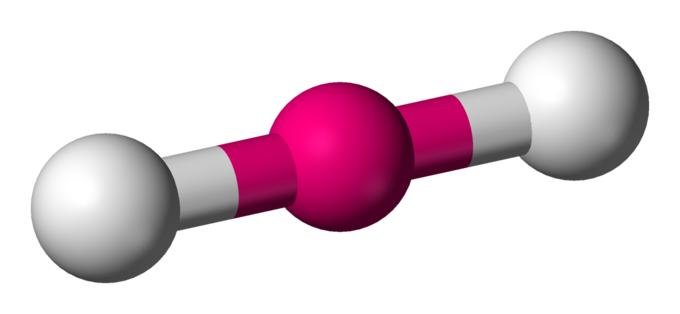Linear electron geometry: This ball-and-stick model represents a linear compound for formula AX2. The two X atoms (in white) are 180° away from one another.

If the central atom also contains one or more pairs of non-bonding electrons, these additional regions of negative charge will behave much like those associated with the bonded atoms. The orbitals containing the various bonding and non-bonding pairs in the valence shell will extend out from the central atom in directions that minimize their mutual repulsions. If the central atom possesses partially occupied d-orbitals, it may be able to accommodate five or six electron pairs, forming what is sometimes called an “expanded octet.”

### Molecular Geometries

Molecular geometries (linear, trigonal, tetrahedral, trigonal bipyramidal, and octahedral) are determined by the VSEPR theory. A table of geometries using the VSEPR theory can facilitate drawing and understanding molecules. The table of molecular geometries can be found in the first figure. The second figure serves as a visual aid for the table.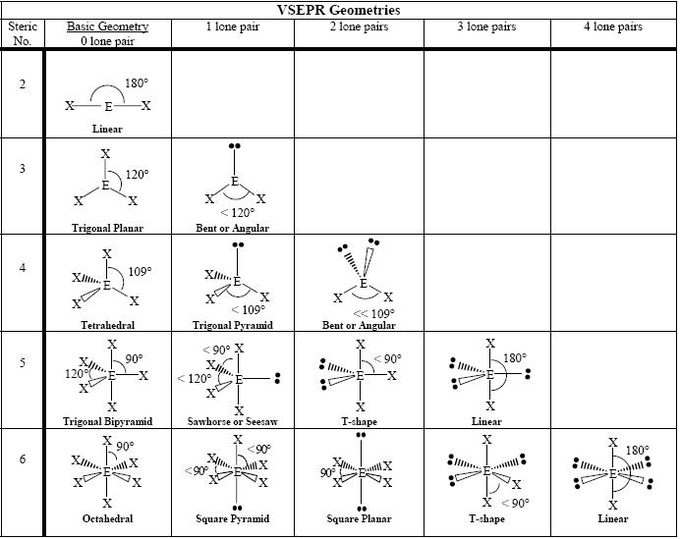VSEPR geometries: A visual guide to molecular geometries using the VSEPR Theory.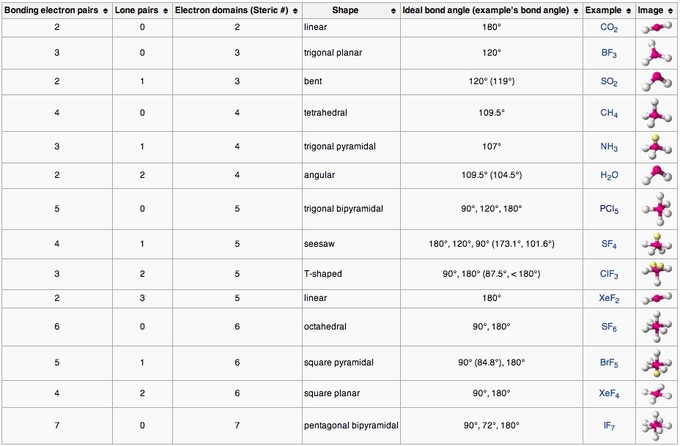VSEPR table of molecular geometries: The bonded angles in the table are ideal angles from the simple VSEPR theory; the actual angle for the example given is in the following column.

How to Determine Molecular Geometry – YouTube: This video describes one method for quickly finding the major geometrical shapes for simple molecules.

## Molecular Geometries

The VSEPR theory describes five main shapes of simple molecules: linear, trigonal planar, tetrahedral, trigonal bipyramidal, and octahedral.

### Learning Objectives

Apply the VSEPR model to determine the geometry of molecules where the central atom contains one or more lone pairs of electrons.

### Key Takeaways

#### Key Points

• Linear: a simple triatomic molecule of the type AX2; its two bonding orbitals are 180° apart.
• Trigonal planar: triangular and in one plane, with bond angles of 120°.
• Tetrahedral: four bonds on one central atom with bond angles of 109.5°.
• Trigonal bipyramidal: five atoms around the central atom; three in a plane with bond angles of 120° and two on opposite ends of the molecule.
• Octahedral: six atoms around the central atom, all with bond angles of 90°.

#### Key Terms

• VSEPR Theory: the Valence Shell Electron Pair Repulsion (VSEPR) model is used to predict the shape of individual molecules based on the extent of electron-pair electrostatic repulsion

### AXE Method

Another way of looking at molecular geometries is through the “AXE method” of electron counting. A in AXE represents the central atom and always has an implied subscript one; X represents the number of sigma bonds between the central and outside atoms (multiple covalent bonds—double, triple, etc.— count as one X); and E represents the number of lone electron pairs surrounding the central atom. The sum of X and E, known as the steric number, is also associated with the total number of hybridized orbitals used by valence bond theory. VSEPR uses the steric number and distribution of X’s and E’s to predict molecular geometric shapes.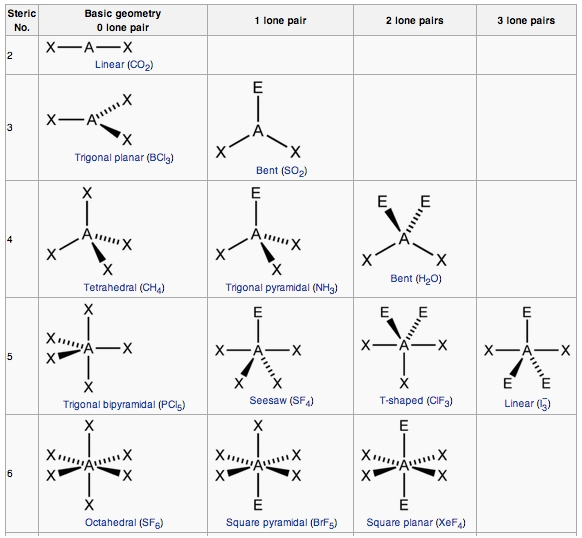AXE method: The A represents the central atom; the X represents the number of sigma bonds between the central atoms and outside atoms; and the E represents the number of lone electron pairs surrounding the central atom. The sum of X and E, known as the steric number, is also associated with the total number of hybridized orbitals used by valence bond theory.

Note that the geometries are named according to the atomic positions only, not the electron arrangement.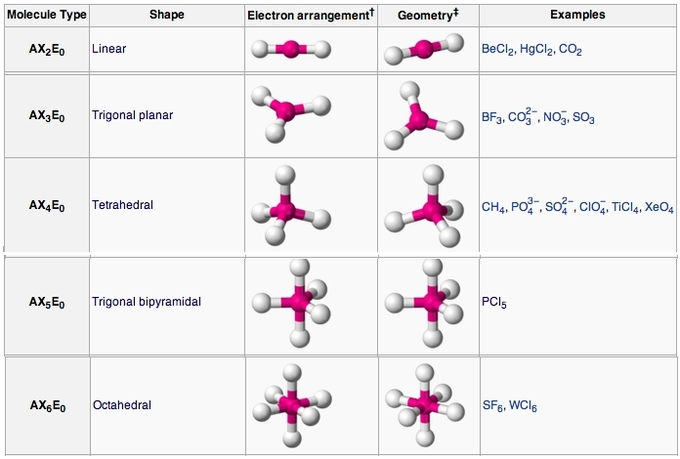AXE method: annotation and examples: AXE annotation, geometry, and examples for each shape.

### Linear

In a linear model, atoms are connected in a straight line, and a bond angle is simply the geometric angle between two adjacent bonds. A simple triatomic molecule of the type AX2 has its two bonding orbitals 180° apart. Examples of triatomic molecules for which VSEPR theory predicts a linear shape include BeCl2 (which does not possess enough electrons to conform to the octet rule) and CO2. When writing out the electron dot formula for carbon dioxide, notice that the C-O bonds are double bonds; this makes no difference to VSEPR theory. The central carbon atom is still joined to two other atoms. The electron clouds that connect the two oxygen atoms are 180° apart.Lewis dot structure of carbon dioxide: Although the central atom (carbon) has four bonds, only two are sigma bonds; it is therefore is represented as AX2E0 in the table.

### Trigonal planar

Molecules with the trigonal planar shape are triangular and in one plane, or flat surface. An AX3 molecule such as BF3 has three regions of electron density extending out from the central atom. The repulsion between these will be at a minimum when the angle between any two is 120o.

### Tetrahedral

Tetra- signifies four, and -hedral relates to a face of a solid; “tetrahedral” literally means “having four faces. ” This shape is found when there are four bonds all on one central atom, with no lone electron pairs. In accordance with the VSEPR theory, the bond angles between the electron bonds are 109.5o. An example of a tetrahedral molecule is methane (CH4). The four equivalent bonds point in four geometrically equivalent directions in three dimensions, corresponding to the four corners of a tetrahedron centered on the carbon atom.The lewis dot structure for methane: The four hydrogen atoms are equidistant from each other, with all bond angles at 109.5°.

A trigonal bipyramidal shape forms when a central atom is surrounded by five atoms in a molecule. In the geometry, three atoms are in the same plane with bond angles of 120°; the other two atoms are on opposite ends of the molecule. Some elements in Group 15 of the periodic table form compounds of the type AX5; examples include PCl5 and AsF5.The Lewis dot structure of phosphorous pentachloride.: The three equatorial atoms are in the same plane, with the two axial atoms located on opposite ends of the molecule.

### Octahedral

Octa- signifies eight, and -hedral relates to a face of a solid, so “octahedral” literally means “having eight faces.” The bond angles are all 90°, and just as four electron pairs experience minimum repulsion when they are directed toward the corners of a tetrahedron, six electron pairs try to point toward the corners of an octahedron. An example of an octahedral molecule (AX6) is sulfur hexafluoride (SF6).

Interactive: Electron Geometry: Molecules assume different shapes due to patterns of shared and unshared electrons. In these examples all electrons affecting the shape of the molecules are shared in the covalent bonds holding the atoms together to form the molecules.

## Lone Electron Pairs

Nonbonding electrons are in orbitals that occupy space, repel the other orbitals, and change a molecule’s shape.

### Learning Objectives

Recognize the effect of lone electron pairs on molecules’ geometries.

### Key Takeaways

#### Key Points

• Orbitals containing the various bonding and nonbonding pairs in the valence shell will extend out from the central atom in directions that minimize their repulsions.
• A nonbonding orbital has no atomic nucleus at its far end to draw the electron cloud toward it; the charge in such an orbital will therefore be concentrated closer to the central atom.
• Nonbonding orbitals exert more repulsion on other orbitals than do bonding orbitals.

#### Key Terms

• coordination number: in chemistry and crystallography, the number of a central atom’s neighbors in a molecule or crystal
• lone pair: a valence set of two electrons that exists without bonding or sharing with other atoms

### Molecular Geometries with Lone Pair Electrons

So far, we have only discussed geometries without any lone pairs of electrons. As you likely noticed in the table of geometries and the AXE method, adding lone pairs changes a molecule ‘s shape. We mentioned before that if the central atom also contains one or more pairs of nonbonding electrons, these additional regions of negative charge will behave much like those associated with the bonded atoms. The orbitals containing the various bonding and nonbonding pairs in the valence shell will extend out from the central atom in directions that minimize their mutual repulsions.AXE method: Lone pairs change a molecule’s shape.

### Coordination Number and the Central Atom

Coordination number refers to the number of electron pairs that surround a given atom, often referred to as the central atom. The geometries of molecules with lone pairs will differ from those without lone pairs, because the lone pair looks like empty space in a molecule. Both classes of geometry are named after the shapes of the imaginary geometric figures (mostly regular solid polygons) that would be centered on the central atom and have an electron pair at each vertex.

In the water molecule (AX2E2), the central atom is O, and the Lewis electron dot formula predicts that there will be two pairs of nonbonding electrons. The oxygen atom will therefore be tetrahedrally coordinated, meaning that it sits at the center of the tetrahedron. Two of the coordination positions are occupied by the shared electron-pairs that constitute the O–H bonds, and the other two by the non-bonding pairs. Therefore, although the oxygen atom is tetrahedrally coordinated, the bonding geometry (shape) of the H2O molecule is described as bent.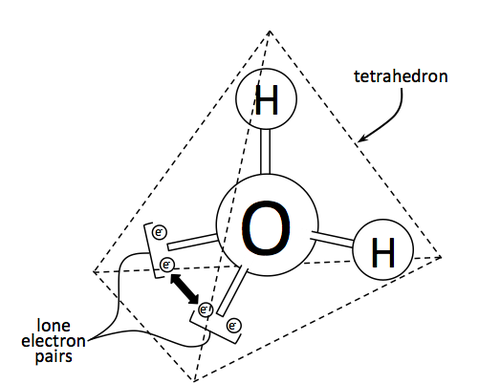The effect of the lone pair on water: Although the oxygen atom is tetrahedrally coordinated, the bonding geometry (shape) of the H2O molecule is described as bent.

### The Repulsive Effect of the Lone Pair Electrons

There is an important difference between bonding and non-bonding electron orbitals. Because a nonbonding orbital has no atomic nucleus at its far end to draw the electron cloud toward it, the charge in such an orbital will be concentrated closer to the central atom; as a consequence, nonbonding orbitals exert more repulsion on other orbitals than do bonding orbitals. In H2O, the two nonbonding orbitals push the bonding orbitals closer together, making the H–O–H angle 104.5° instead of the tetrahedral angle of 109.5°.

The electron-dot structure of NH3 places one pair of nonbonding electrons in the valence shell of the nitrogen atom. This means that there are three bonded atoms and one lone pair for a coordination number of four around the nitrogen, the same as occurs in H2O.The Lewis dot structure for ammonia, NH3.: The lone pair attached to the central nitrogen creates bond angles that differ from the tetrahedral 109.5 °.

We can therefore predict that the three hydrogen atoms will lie at the corners of a tetrahedron centered on the nitrogen atom. The lone pair orbital will point toward the fourth corner of the tetrahedron, but since that position will be vacant, the NH3 molecule itself cannot be tetrahedral; instead, it assumes a pyramidal shape, more specifically, that of a trigonal pyramid (a pyramid with a triangular base). The hydrogen atoms are all in the same plane, with the nitrogen outside of the plane. The non-bonding electrons push the bonding orbitals together slightly, making the H–N–H bond angles about 107°.

In 5-coordinated molecules containing lone pairs, these non-bonding orbitals (which are closer to the central atom and thus more likely to be repelled by other orbitals) will preferentially reside in the equatorial plane. This will place them at 90° angles with respect to no more than two axially-oriented bonding orbitals. We can therefore predict that an AX4E molecule (one in which the central atom A is coordinated to four other atoms X and to one nonbonding electron pair) such as SF4 will have a “see-saw” shape.Example of a see-saw structure: Try to imagine this molecule teetering on each end, and you will have a visual representation of a see-saw.

Substituting nonbonding pairs for bonded atoms reduces the triangular bipyramid coordination to even simpler molecular shapes.

Interactive: Unshared Electrons and the “Bent” Shape: Use the 3D model to see how unshared electrons repel those that are shared in the bonds between hydrogen and oxygen, causing the molecule to have a “bent” shape.